# How I found the first modified Dirichlet kernel in 5D numbers.

Back in Jan 2014 I was able to solve the circular and complex 5D numbers systems using the so called ‘tau-calculus’. Basically tau-calculus is very easy to understand:

1. Find a basis vector (from an imaginary number) that has determinant +1.
2. Craft analytical expressions that you cannot solve but use internet applets for numerical answers. Or, equivalent:
3. Use an applet to calculate the logarithm of your basis vector once you have put it on a matrix representation.
4. Start thinking long and hard until you have solved the math analysis…

This is easy to understand but I could only do this in three and five dimensional space, seven or eleven dimensional space? It is now 2.5 years later and I still have no clue whatsoever.

Anyway back in the time it was a great victory to find the exponential curves in 5D space. May be some people with insect like minds think that the Euler identity is the greatest formula ever found but let me tell you:
The more of those exponential circles and curves you find, the more boring the complex plane becomes…

In the months after Jan 2014 I wanted to understand the behavior of the coordinate functions that come along those two exponential curves. But the problem kept elusive until I realized I had one more round of internet applets waiting for me.
I had to feed this internet applet for the log of a matrix over 50 matrices and write down the answers it gave me; that was a lot of work because every matrix had 25 entries.

Originally I planned for ‘digesting’ 10 matrices a day so it would be a five day project, but when I finally started somewhere in June 2014 after two days I was ready to sketch my strongly desired coordinate function of the very first coordinate in 5D space.

I still remember sitting at my table and do the drawing and when finished it was so fast that I realized the next: This is a Dirichlet kernel.

And the way I used it, it was so more simple to write down this kernel and if you think how those exponential curves in higher dimensional spaces work with their starting coordinate function and all the time lags that follow… This finding will forever be in the top ten of most perfect math found by your writer Reinko Venema…

__________

Ok, what is in this new post?
Nine pictures of size 550 x 775 pixels containing:
Basic definitions upon 5D complex and circular number &
Tau calculus for the exponential curve &
Explaining how I found the graph of the first modified Dirichlet kernel &
A small quantum physics example related to probability amplitudes &
Some cute integrals that are easy to crack now.

Hope you can learn a bit from it, do not worry if you do not understand all details because even compared to the 2D complex plane or the 3D complex numbers this website is about:
The 5D realm is a space on it’s own!

Have fun reading & thinking upon it: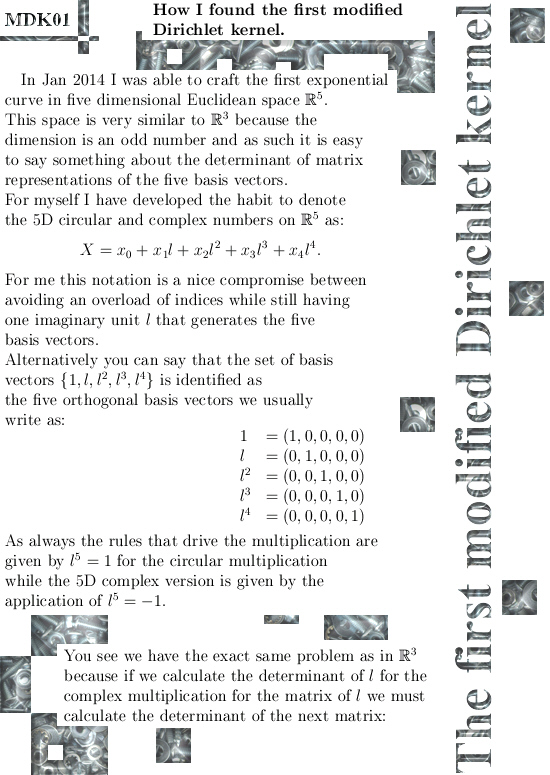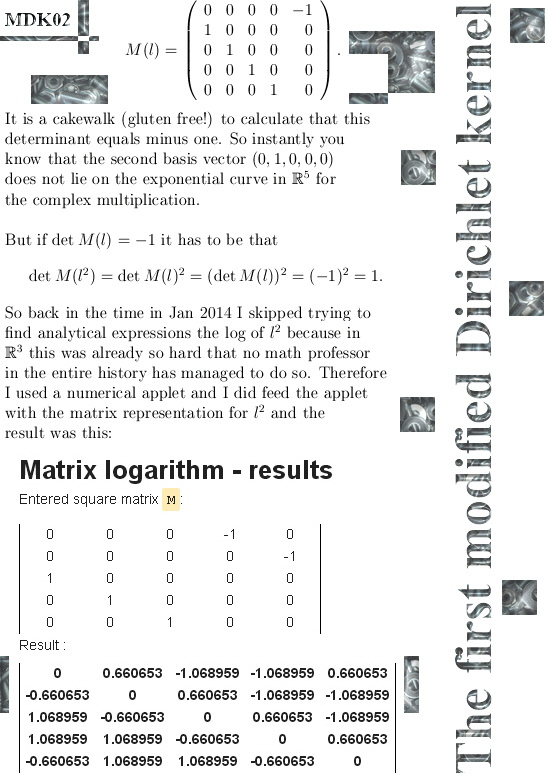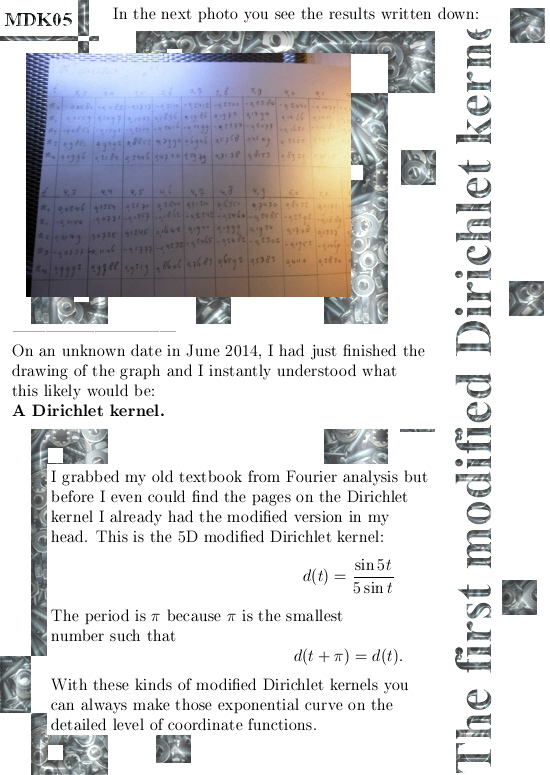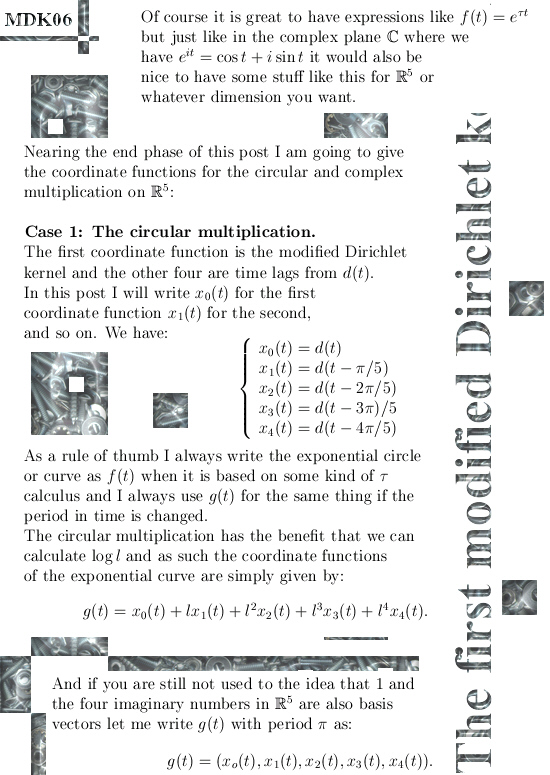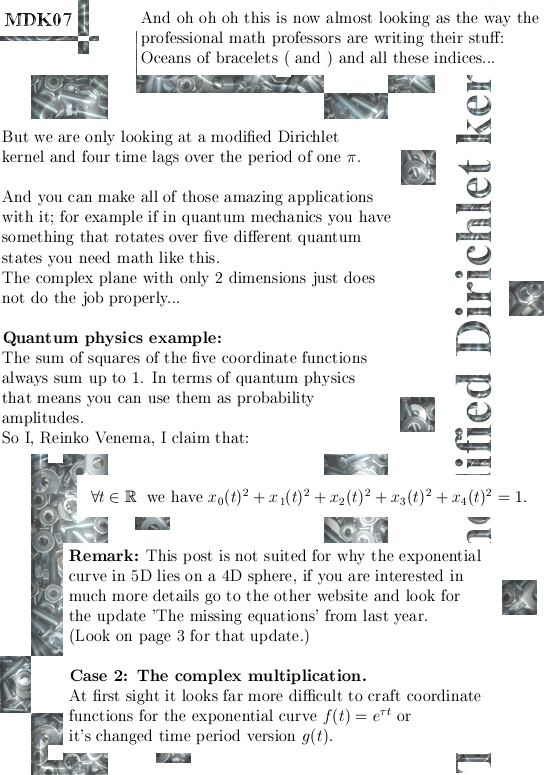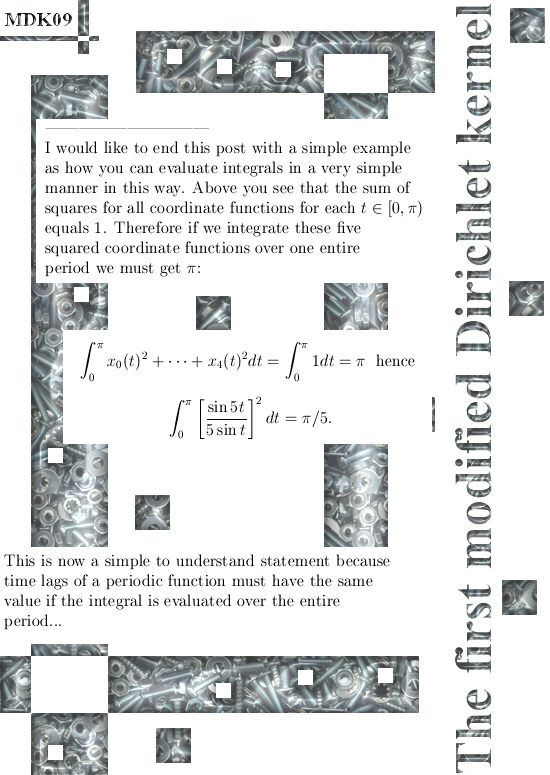A few links of interest are the next:

You need a good applet for the log of a matrix representation if you want, for example, crack the open problem in tau calculus for the 7D complex numbers:
http://calculator.vhex.net/calculator/linear-algebra/matrix-logarithm

My original update on the other website about the 5D number systems from Jan 2014:
http://kinkytshirts.nl/rootdirectory/just_some_math/3d_complex_stuff02.htm#30Jan2014

If you are more interested in those kind of weird looking integrals suddenly easy to solve if you use a proper combination of geometry and analysis, the update from July 2015 upon the missing equations is also worth a visit:
The missing equations.
http://kinkytshirts.nl/rootdirectory/just_some_math/3d_complex_stuff03.htm#14July2015

__________

This is what I more or less had to say, have a nice life or try to get one.
See you in the next post.